# Samacheer Kalvi 9th Maths Book Solutions Chapter 6 | Trigonometry Answers For Tamil Nadu Board

Samacheer Kalvi 9th Maths Book Solutions Chapter 6 – Trigonometry is available here. The Samacheer Kalvi 9th Maths book answers of Chapter 6, available at BYJU’S, contain step by step explanations designed by our mathematics experts. All these important questions are based on the new pattern prescribed by the Tamil Nadu board. Students can also get the solutions of other chapters on Samacheer Kalvi 9th Maths solutions. They can solve these Samacheer Kalvi Class 9 Maths Book Chapter 6 Quesitons with Solutions for practise, referring to the solutions later to analyse the answers.

## Samacheer Kalvi Class 9 Maths Book Chapter 6 Questions and Solutions

Chapter 6 of the Samacheer Kalvi 9th Maths guide will help the students to solve problems related to trigonometric ratios, trigonometric ratios of some special angles, trigonometric ratios for complementary angles, method of using a trigonometric table.

### Samacheer Kalvi 9th Maths Chapter 6: Trigonometry Book Exercise 6.1 Questions and Solutions

Question 1: From the given figure, find all the trigonometric ratios of angle B.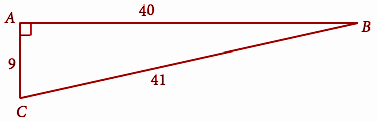Solution:

From the triangle ABC,

Hypotenuse (BC) = 41

Opposite (AC) = 9

sin B = Opposite / Hypotenuse

sin B = AC / BC = 6 / 41

cos B = Adjacent / Hypotenuse

cos B = AB / BC = 40 / 41

tan B = Opposite / Adjacent

tan B = AC / AB = 6 / 40

cosec B = Hypotenuse / Opposite

cosec B = BC / AC = 41 / 6

sec B = Hypotenuse / Adjacent

sec B = BC / AB = 41 / 40

cot B = Adjacent / Opposite

cot B = AB / AC = 40 / 6

Question 2: From the given figure, find the values of

(i) sin B

(ii) sec B

(iii) cot B

(iv) cos C

(v) tan C

(vi) cosec C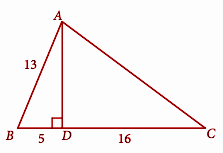Solution:

In ▵ABD,

AC2 = 122 + 162

AC2 = 144 + 256

AC = 20

(i) sin B = AD / AB

sin B = 12 / 13

(ii) sec B = AB / BD

sec B = 13 / 5

(iii) cot B = BD / AD

cot B = 5 / 12

(iv) cos C = DC / AC

cos C = 16 / 20 = 4 / 5

(v) tan C = AD / DC

tan C = 12 / 16 = 3 / 4

(vi) cosec C = AC / AD

cosec C = 20 / 12 = 5 / 3

Question 3: If 2 cosΘ = √3, then find all the trigonometric ratios of angle Θ.

Solution:

cos θ = √3 / 2 = Adjacent / Hypotenuse

(Opposite)2 = 22 – (√3)2 = 4 – 3

Opposite = 1

sin A = Opposite / Hypotenuse = 1 / 2

cos A = Adjacent / Hypotenuse = √3 / 2

tan A = Opposite / Adjacent = 1 / √3

cosec A = Hypotenuse / Opposite = 2 / 1

sec A = Hypotenuse / Adjacent = 2 / √3

cot A = Adjacent / Opposite = √3 / 1

Question 4: If cos A = 3 / 5, then find the value of (sin A – cos A) / 2 tan A

Solution:

cos A = 3/5 = Adjacent side / Hypotenuse side

(Opposite)2 = (Hypotenuse)2 – (Adjacent)2 = 52 – 32

(Opposite)2 = 25 – 9 = 16

Opposite = √16 = 4

sin A = opposite / hypotenuse = 4 / 5

tan A = opposite / adjacent

tan A = 4 / 3

(sin A – cos A) / 2 tan A = (4 / 5) – (3 / 5) / [2 (4 / 3)]

= (1 / 5) / (8 / 3)

= 3 / 40

Question 5: If cos A = (2x) / (1 + x2), then find the values of sin A and tan A in terms of x.

Solution:

cos A = 2x / (1 + x2) = Adjacent / Hypotenuse

Opposite2 = (1 + x2)2 – (2x)2

= 1 + x4 + 2x2 – 4x2

= 1 + x4 – 2x2

= (1 – x2)2

Opposite = 1 – x2

sin A = Opposite / Hypotenuse

sin A = (1 – x2) / (1 + x2)

tan A = Opposite / Adjacent

tan A = (1 – x2) / 2x

Question 6: If sin θ = a / √a2 + b2, then show that b sin θ = a cos θ

Solution:

Given that, sin θ = a / √a2 + b2

sin θ = Opposite / Hypotenuse

(Adjacent)2 = (√a2 + b2)2 – (a)2

b sin θ = ab / √a2 + b2 —-(1)

cos θ = b / √a2 + b2

a cos θ = ab / √a2 + b2 —-(2)

(1) = (2)

Hence proved.

Question 7: If 3 cot A = 2, then find the value of (4 sin A – 3 cos A) / (2 sin A + 3 cos A)

Solution:

cot A = 2 / 3 = Adjacent / Opposite

(Hypotenuse)2 = 32 + 22

Hypotenuse = √13

sin A = Opposite / Hypotenuse

sin A = 3 / √13

cos A = Adjacent / Hypotenuse

cos A = 2 / √13

(4 sin A – 3 cos A) / (2 sin A + 3 cos A) :

= [4 (3 / √13) – 3 (2 / √13)] / [2 (3 / √13) + 3 (2 / √13)]

= [(12 – 6) / √13] / [(6 + 6) / √13]

= 6 / 12

= 1 / 2

Question 8: If cos θ:sin θ = 1:2, then find the value of (8 cos θ – 2 sin θ) / (4 cos θ + 2 sin θ).

Solution:

cos θ : sin θ = 1 : 2

cos θ / sin θ = 1 / 2

cot θ = 1 / 2 = Adjacent / Opposite

(Hypotenuse)2 = (Opposite)2 + (Adjacent)2 = 12 + 22

(Hypotenuse)2 = 5

Hypotenuse = √5

sin θ = 1 / √5, cos θ = 2 / √5

(8 cos θ – 2 sin θ) / (4 cos θ + 2 sin θ)

= (8 / √5) – (4 / √5) / (4 / √5) + 2 (2 / √5)

= (4 / √5) / (8 / √5)

= 4 / 8

= 1 / 2

Question 9: From the given figure, prove that θ + Φ = 90°. Also, prove that there are two other right-angled triangles. Find sin a, cos β and tan Φ.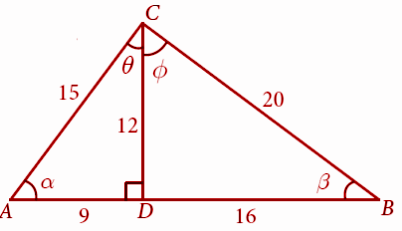Solution:

In △ACD,

sin a = Opposite / Hypotenuse

sin a = DC / AC

sin a = 12 / 15 = 4 / 5

In △BDC,

cos β = Adjacent / Hypotenuse = DB / BC

cos β = 16 / 20 = 4 / 5

tan Φ = Opposite / Adjacent

tan Φ = DB / DC = 16 / 12 = 4 / 3

Question 10: A boy standing at a point O finds his kite flying at a point P with distance OP = 25m. It is at a height of 5m from the ground. When the thread is extended by 10m from P, it reaches a point Q. What will be the height QN of the kite from the ground? (use trigonometric ratios)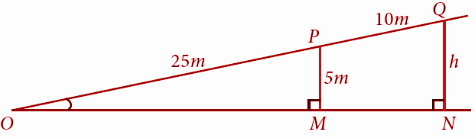Solution:

In △OPM,

tan θ = PM / OP = 5 / 25 —(1)

tan θ = ON / (OP + PQ)

= h / (25 + 10)

= h / 35 —(2)

(1) = (2)

5 / 25 = h / 35

(1 / 5) (35) = h

h = 7

### Samacheer Kalvi 9th Maths Chapter 6: Trigonometry Book Exercise 6.2 Questions and Solutions

Question 1: Verify the following equalities:

[i] sin260°+ cos260° = 1

[ii] 1 + tan230° = sec230°

[iii] cos 90° = 1 – 2 sin245° = 2 cos245° – 1

[iv] sin 30° cos 60° + cos 30° sin 60° = sin 90°

Solution:

[i] sin260° + cos260°

= (√3 / 2)2 + (1 / 2)2

= (3 / 4) + (1 / 4)

= (3 + 1) / 4

= 4 / 4

sin260° + cos260° = 1

[ii] 1 + tan230° = sec230°

1 + tan230° = 1 + (1 / √3)2

1 + tan230° = 1 + (1 / 3)

1 + tan230° = 4 / 3 —– (1)

sec230° = (2 / √3)2

sec230° = 4 / 3 —– (2)

From (1) and (2), we get

1 + tan230° = sec230°

[iii] cos 90° = 1 – 2 sin245° = 2 cos245° – 1

cos 90° = 0 —– (1)

1 – 2 sin245° = 1 – 2 (1 / √2)2

1 – 2 sin245° = 1 – 2 (1 / 2)

1 – 2 sin245° = 0 —– (2)

2 cos2 45° – 1 = 2 (1 / √2)2 – 1

2 cos2 45° – 1 = 2 (1 / 2) – 1

2 cos2 45° – 1 = 0 ——-(3)

From (1), (2) and (3),

cos90° = 1 – 2 sin245° = 2 cos245° – 1

[iv] sin 30° cos 60° + cos 30° sin 60° = sin 90°

sin 30° cos 60° + cos 30° sin 60°

= (1 / 2) (1 / 2) + (√3 / 2) (√3 / 2)

= (1 / 4) + (3 / 4)

= (1 + 3) / 4

= 4 / 4

= 1 —– (1)

sin 90° = 1 —– (2)

From (1) and (2),

sin 30° cos 60° + cos 30° sin 60° = sin 90°

Question 2: Find the value of the following:

[i] (tan45° / cosec30°) + (sec60° / cot45°) – (5 sin90° / 2 cos0°)

[ii] (sin 90°+ cos 60°+ cos 45°) × (sin 30°+ cos 0°- cos 45°)

[iii] sin230° – 2 cos360° + 3 tan445°

Solution:

[i] (tan45° / cosec30°) + (sec60° / cot45°) – (5 sin90° / 2 cos0°)

(tan45° / cosec30°) + (sec60° / cot45°) – (5 sin90° / 2 cos0°)

= (1 / 2) + (2 / 1) – (5 (1) / 2 (1))

= (1 / 2) + (4 / 2) – (5 / 2)

= (1 + 4 – 5) / 2

= 0

[ii] (sin 90°+ cos 60°+ cos 45°) × (sin 30°+ cos 0°- cos 45°)

(sin 90°+ cos 60°+ cos 45°) × (sin 30°+ cos 0°- cos 45°)

= [1 + (1 / 2) + (1 / √2)] × [(1 / 2) + 1 – (1 / √2)]

= [(3 / 2) + (1 / √2)] × [(3 / 2) – (1 / √2)]

= [(3 / 2)2 – (1 / √2)2]

= [(9 / 4) – (1 / 2)]

= (9 – 2) / 4

= 7 / 4

[iii] sin230° – 2 cos360° + 3 tan445°

sin230° – 2 cos360° + 3 tan445°

= (1 / 2)2 – 2 (1 / 2)3 + 3 (1)4

= (1 / 4) – (1 / 4) + 3

= 3

Question 3: Verify: cos 3A = 4 cos3A – 3 cos A , when A = 30°

Solution:

cos 3A = cos 3 (30°)

cos 3A = cos 90°

cos 3A = 0 — (1)

4 cos3A – 3 cosA

= 4 cos330° – 3 cos 30°

= 4 (√3 / 2)3 – 3 (√3 / 2)

= (12√3 / 8) – (3√3 / 2)

= (3√3 / 2) – (3√3 / 2)

= 0 —–(2)

From (1) and (2),

cos 3A = 4 cos3A – 3 cos A, when A = 30°.

Question 4: Find the value of 8sin 2x cos 4x sin6x, when x = 15°.

Solution:

8 sin 2x cos 4x sin6x :

= 8 sin 2 (15°) cos 4 (15°) sin 6 (15°)

= 8 sin 30° cos 60° sin 90°

= 8 (1 / 2) (1 / 2) (1)

= 2

### Samacheer Kalvi 9th Maths Chapter 6: Trigonometry Book Exercise 6.3 Questions and Solutions

Question 1: Find the value of:

[i] (cos 47 / sin 43)2 + (sin 72 / cos 18)2 – 2cos2 45

[ii] (cos 70 / sin 20)2 + (cos 59 / sin 31)2 + cos θ / sin (90 – θ) – 8 cos2 60

[iii] tan 15° tan 30° tan 45° tan 60° tan 75°

[iv] [cot θ / tan (90 – θ)] + [cos (90 – θ) tan θ sec (90 – θ) / sin (90 – θ) cot (90 – θ) cosec (90 – θ)]

Solution:

= (cos 47 / sin 43)2 + (sin 72 / cos 18)2 – 2cos2 45

cos 47 = sin (90 – 47) = sin 43

sin 72 = cos (90 – 72) = cos 18

cos 45 = 1 / √2

= (sin 43 / sin 43)2 + (cos 18 / cos 18)2 – 2 (1 / √2)2

= 12 + 12 – 1

= 1

[ii] (cos 70 / sin 20)2 + (cos 59 / sin 31)2 + cos θ / sin (90 – θ) – 8 cos2 60

= (cos 70 / sin 20)2 + (cos 59 / sin 31)2 + cos θ / sin (90 – θ) – 8 cos2 60

cos 70 = sin (90 – 70) = sin 20

cos 59 = sin (90 – 59) = sin 31

sin (90 – θ) = cos θ

cos2 60 = (1 / 2)2 = 1/4

= (sin 20 / sin 20)2 + (sin 31 / sin 31)2 + (cos θ / cosθ) – 8 (1 / 4)

= 1 + 1 + 1 – 2

= 3 – 2

= 1

[iii] tan 15° tan 30° tan 45° tan 60° tan 75°

= tan 15° tan 30° tan 45° tan 60° tan 75°

tan 75° = cot (90 – 15) = cot 15

tan 30° = 1 / √3, tan 45° = 1, tan 60° = √3

= tan 15° (1 / √3) 1 (√3) cot 15°

= tan 15° (1 / tan 15°)

= 1

[iv] [cot θ / tan (90 – θ)] + [cos (90 – θ) tan θ sec (90 – θ) / sin (90 – θ) cot (90 – θ) cosec (90 – θ)]

= [cot θ / tan (90 – θ)] + [cos (90 – θ) tan θ sec (90 – θ) / sin (90 – θ) cot (90 – θ) cosec (90 – θ)]

= [cot θ / cot θ] + [sin θ tan θ cosec θ / cos θ tan θ sec θ]

= 1 + (sin θ cosec θ / cos θ sec θ)

= 1 + 1

= 2

### Samacheer Kalvi 9th Maths Chapter 6: Trigonometry Book Exercise 6.4 Questions and Solutions

Question 1: Find the value of the following:

[i] sin 49°

[ii] cos 74° 39′

[iii] tan 54° 26′

[iv] sin 21° 21′

[v] cos 33° 53′

[vi] tan 70° 17′

Solution:

(i) sin 49°

sin 49° = 0.7547

(ii) cos 74° 39′

cos 74° 39′

= cos 74° 36′ + 3′

= 0.2656 – 0.008

= 0.2648

(iii) tan 54° 26′

tan 54° 26′

= tan 54° 24′ + 2”

= 1.3968 + 0.0017

= 1.3985

(iv) sin 21° 21′

sin 21° 21′

= sin 21° 18′ + 3”

= 0.3633 + 0.0008

= 0.3641

(v) cos 33° 53′

cos 33° 53′

= cos 33° 48′ – 5”

= 0.8310 – 0.0008

= 0.8302

(vi) tan 70° 17′

tan 70° 17′

= tan 70° 12′ + 5”

= 2.2776 + 0.0131

= 2.2907

Question 2: Find the value of θ if

(i) sin θ = 0.9975

(ii) cos θ = 0.6763

(iii) tan θ = 0.0720

(iv) cos θ = 0.0410

(v) cos θ = 7.5958

Solution:

(i) sin θ = 0.9975

θ = sin 85° 57′

(ii) cos θ = 0.6763

θ = sin 47° 27′

(iii) cos θ = 0.6763

θ = sin 4° 7′

(iv) cos θ = 0.0410

θ = sin 87° 39′

(v) cos θ = 7.5958

θ = cos 82° 30′

Question 3: Find the value of the following:

(i) sin 65° 39′ + cos 24° 57′ + tan 10° 10′

(ii) tan 70° 58′ + cos 15° 26′ – sin 84° 59′

Solution:

(i) sin 65° 39′ + cos 24° 57′ + tan 10° 10′

sin 65° 39′ = sin 65° 36′ + 3”

= 0.9107 + 0.0004

= 0.9111

cos 24° 57′ = 0.9070 – 0.0004

= 0.9066

tan 10° 10′ = 0.1781 + 0.0012

= 0.1793

sin 65°39’+cos 24°57’+tan 10°10′

= 0.9111 + 0.9066 + 0.1793

= 1.997

(ii) tan 70° 58′ + cos 15° 26′ – sin 84° 59′

tan 70° 58′ = tan 70° 54′ + 4”

= 2.8878 + 0.0104

= 2.8982

cos 15° 26′

= cos 15° 24′ – 2”

= 0.9641 – 0.0004

= 0.9637

sin 84° 59′ = sin 84° 54′ + 5”

= 0.9960 + 0.0002

= 0.9962

tan 70° 58′ + cos 15° 26′ – sin 84° 59′

= 2.8982 + 0.9637 – 0.9962

= 2.8657

Question 4: Find the area of a right triangle whose hypotenuse is 10cm and one of the acute angles is 24o24’.

Solution:

Hypotenuse of triangle = 10cm

Since it is a right angled triangle, the trigonometry identities can be applied.

Area of triangle = (1 / 2) * height * base

Find the height

sin Ф = height / hypotenuse

sin 24 = height / 10

height = 4.07 cm

Find the base

cos Ф = base / hypotenuse

cos 24 = base / 10

base = 9.135 cm

area of the triangle = (1 / 2) * (4.07) * (9.135)

= 18.6 cm²

Question 5: Find the angle made by a ladder of length 5m with the ground, if one of its ends is 4m away from the wall and the other end is on the wall.

Solution:

The ladder leaning on the wall forms a right-angled triangle.

Let the sides of the formed right-angled triangle be a, b and c.

a = height = the height of the wall

b = base = the distance between the ladder and the wall

c = the hypotenuse = the length of the ladder

Here,

C = 5m

b = 4m

Let the angle made by the ladder with the ground be O.

Since b is the adjacent side and c is the hypotenuse side, cosine is used.

Cos O = adjacent side / hypotenuse side

Cos O = 4 / 5

= 0.8

O = cos-1 of 0.8

= 40.97°

The angle that the ladder makes with the ground is 40.97°.

Question 6: In the given figure, HT shows the height of a tree standing vertically. From a point P, the angle of elevation of the top of the tree (that is ∠P ) measures 42° and the distance to the tree is 60 metres. Find the height of the tree.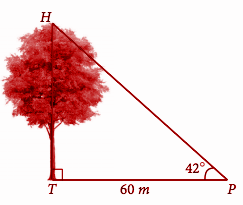Solution:

To find the value of length HT.

Let the length of the tree be x.

tan 42o = HT / HP

0.900 = x / 60

x = 54

x = 54 meters